Module 3.3 Notes "Model Building Process"

 Index to Module Three Notes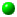3.1: Introduction to Multiple Regression3.2: Curvature, Dummy Variables and Interaction3.3: Model Building Process

To recap, multiple regression allows us to study the relationship between a dependent variable and multiple independent variables. The independent variables can be numerical (quantitative) or dummy (qualitative or categorical) variables. They can also be functions of independent variables, such as the curvature component and/or the interaction component. So how do we know what component to put into the multiple regression model?

You could always use trial and error - but that isn't good science. Formal techniques include forward selection (start with a simple linear regression model and add predictor variables as long as significant improvement is made); backward selection (start with a large pool of predictor variables and successively remove variables that do not significantly contribute to the prediction); stepwise regression (like forward selection but variables once added are also tested for removal after subsequent addition of more variables to determine if better models result); and best subsets (create subsets or combinations of all predictor variables and select the model with best statistical and practical utility). The Handbook of Parametric and Non parametric Statistical Procedures, by David Sheskin provides more details on the various methods.

The approach I would like you to use in Assignment 3 is a modification of the backward selection method. We will hypothesis a full multiple regression model with a quantitative independent variable, qualitative independent variable, curvature and interaction. We will then start taking components away that do not contribute to the statistical utility of the model. However, the way we take components away will be by following a decision tree in order to give us some structure.

In getting ready for Assignment 1, the first item is to enter your data into an Excel Spreadsheet, and create the curvature and interaction terms. I will demonstrate how to do this with several examples later in these notes. Item 2 requires that you build and test at least 2 of the hypothesized models shown in the next section, starting with Model 1 (Item 3 of Assignment 3).

Hypothesized Models

The decision tree will "walk us through" the selection of one of the following seven models that best fits the sample of data for your Assignment 3. The symbol QN stands for your quantitative X, QN2 represents curvature, QL represents the qualitative X, and QN*QL represents interaction.

Model 1: E(Y) = B0 + B1 QN + B2 QN2 + B3 QL + B4 QN*QL

Model 2: E(Y) = B0 + B1 QN + B3 QL + B4 QN*QL

Model 3: E(Y) = B0 + B1 QN + B2 QN2 + B3 QL

Model 4: E(Y) = B0 + B1 QN + B2 QN2

Model 5: E(Y) = B0 + B1 QN + B3 QL

Model 6: E(Y) = B0 + B3 QL

Model 7: E(Y) = B0 + B1 QN

A word description for each model is that the hypothesized regression model for predicting Y includes:

Model 1: Quantitative and qualitative variables, curvature and interaction

Model 2: Quantitative and qualitative variables, and interaction

Model 3: Quantitative and qualitative variables, and curvature

Model 4: A quantitative variable and curvature

Model 5: Quantitative and qualitative variables

Model 6: A qualitative variable

Model 7: A quantitative variable

Please note that there is no curvature term for the qualitative variable since categorical variables have no number properties beyond their 0/1 designation. To determine which model is best your sample data, we use the following decision tree.

Model Building Decision Tree

Item 3 in Assignment 3 asks you to run the Model 1 Regression Model. Item 4 in Assignment 3 requires that you first test to determine if curvature is important in that model. The decision tree then provides the actions you follow depending on the outcome of that and subsequent tests.

Item 4. Test curvature (Model 1 vs. 2).
A. If curvature is significant, test interaction (Model 1 vs. 3).
(1). If interaction is significant, stop. Model 1 is "best" model. Go to Item 5 (obtaining the residual, normal and line fit plots).

(2). If interaction is not significant, Build Model 3 and test QL (Model 3 vs. 4).

a. If QL is significant, stop. Model 3 is "best" model. Go to Item 5.

b. If QL is not significant, build Model 4 and stop. Model 4 is "best" model. Go to Item 5.

B. If curvature is not significant, build Model 2 and test interaction (Model 2 vs. 5).

(1). If interaction is significant, stop. Model 2 is "best" model. Go to item 5.

(2). If interaction is not significant, build Model 5 and test QL (Model 5 vs. 7).

a. If QL is significant, test QN (Model 5 vs. 6).
1. If QN is significant, stop. Model 5 is "best" model. Go to Item 5.

2. If QN is not significant, stop. Model 6 is "best model. Go to Item 5.

b. If QL is not significant, stop and select Model 7 as "best" model, even if the Model is not significant. Go to Item 5.

Although Item 5 is not part of the decision tree, it is part of the Assignment 3 requirement so I am repeating it here for ready reference (it and the Item 4 decision tree appear in the Main Module 3 Web Page).

5. Rerun the data analysis regression tool for your "best" model, and include and be able to describe or interpret the following printouts:
• Residual plot :
• QN (Model 4 or 7)
• QL Model 6)
• QN and QL (Models 1, 2, 3, or 5)
• Normal probability plot (for all Models)
• Fitted Line Plot:
• QN2 (Models 1, 3, 4)
• QN (Models 1, 2, 3, 4, 5, 7)
• QL (Models 1, 2, 3, 5, 6)

Example One: Drug Effectiveness

Item 1: Enter Data
I am going to use as my first example, the study introduced at the end of Module Notes 3.2: the new drug effectiveness study data.

This example concerned an experiment done by an over-the-counter drug manufacturer interested in testing a new drug. This drug is designed to be effective in curing an illness most commonly found in older patients. The dependent variable is Effect, which stands for the effectiveness of recovery from a certain illness. It is measured on a scale of 0 to 100 (100 is more effective). The quantitative independent variable is age, and the qualitative independent variable is whether or not the new drug was present in the experiment (drug = 0) or absent (subjects took the old drug, drug = 1) in the experiment. Note that half of the subjects were given the new drug, and half were given the old drug (they were not told which one to avoid bias in the experiment).

Worksheet 3.3.1 shows the data entry (Item 1, Assignment 1).

Worksheet 3.3.1
 Age Age^2 Drug Age*Drug Effect 21 441 1 21 56 19 361 0 0 28 28 784 1 28 55 23 529 0 0 25 67 4489 0 0 71 33 1089 1 33 63 33 1089 1 33 52 56 3136 0 0 62 45 2025 0 0 50 38 1444 1 38 58 37 1369 0 0 46 27 729 0 0 34 43 1849 1 43 65 47 2209 0 0 59 48 2304 1 48 64 53 2809 1 53 61 29 841 0 0 36 53 2809 1 53 69 58 3364 1 58 73 63 3969 1 63 62 59 3481 0 0 71 51 2601 0 0 62 67 4489 1 67 70 63 3969 0 0 71

I entered the quantitative X, Age, in the first column. The second column is the curvature term which is constructed by squaring each value in the Age Column. The third column is the qualitative X, drug (0 = new drug was present, 1 = new drug was absent). The fourth column includes the interaction component, which is obtained by multiplying the respective cells in the Age Column times the cells in the Drug Column. I titled this Age*Drug to represent the multiplication. Finally, the last column contains the quantitative Y variable.

You are free to set up your data as you wish, the above format seems to be most efficient of the various formats I tried. Your requirement is similar to the example above: one quantitative X, one qualitative X, and a quantitative Y. Curvature and interaction are constructed.

Item 2: Build Model 1
Item 2, Assignment 3, requires that you hypothesize and run a full model with the quantitative and qualitative variables, curvature and interaction.

Worksheet 3.3.2 illustrates the regression summary from using the Regression Data Analysis Add In.

Worksheet 3.3.2
 SUMMARY OUTPUT Regression Statistics Multiple R 0.967077619 R Square 0.935239122 Adjusted R Square 0.921605253 Standard Error 3.888421114 Observations 24 ANOVA df SS MS F Significance F Regression 4 4148.681777 1037.170444 68.59675111 5.04008E-11 Residual 19 287.2765564 15.11981876 Total 23 4435.958333 Coefficients Standard Error t Stat P-value Lower 95% Upper 95% Intercept -0.05567568 7.441829412 -0.007481451 0.994108718 -15.63160849 15.52025713 Age 1.372995238 0.36799282 3.731038117 0.001415911 0.602777175 2.143213302 Age^2 -0.003978725 0.004228799 -0.940864063 0.358583821 -0.012829706 0.004872256 Drug 40.47038187 5.11462738 7.912674543 1.97194E-07 29.7653404 51.17542333 Age*Drug -0.688569563 0.109012691 -6.316416545 4.6164E-06 -0.916735817 -0.460403308

Note: I do not recommend selecting any of the output options at this point. The Regression Summary is all that we need for the various component test procedures in the decision tree. Once we have our best model, we can rerun it and produce all of the output options such as residual, normal and line fit plots.

First Test: Curvature
Item 4, Assignment 3, requires that we test curvature. This is where the decision tree begins. Since we have the full Model 1 constructed, we can easily test for curvature by comparing Model 1 to Model 2. Model 2 is just like Model 1 except it doesn't have curvature.

The slope coefficient that we need to use to test curvature is B2. The null and alternate hypotheses to test curvature are:

H0: B2 = 0 (curvature is not important)
Ha: B2 =/= 0 (curvature is important)

The hypothesized model associated with the null hypothesis at this point in the decision tree is Model 2, and the hypothesized model associated with the alternative hypothesis is Model 1:

Model 2 Associates with H0: Effect = B0 + B1 Age + B3 Drug +
B4 Age*Drug

Model 1 Associates with H1: Effect = B0 + B1 Age + B2 Age2 +

B3Drug + B4 Age*Drug

Since the p-value (0.35858) for the curvature term (Age^2) in Worksheet 3.3.2 is greater than alpha of 0.01, do not reject the null hypothesis, and conclude that curvature is not important. This means that Model 2 is a better predictor than Model 1. We need to rerun the regression without the curvature term, and next test interaction. This is noted in Decision Tree Action B.

Second Test: Interaction
To create a sample regression model for hypothesized Model 2, I remove the curvature column, and redo the regression analysis. The practice I follow is to make a copy of the original data to another worksheet tab or area on the current tab, and then delete the curvature column (or curvature data if I need information below the data in that column). If you delete the data, you need to shift the remaining data (qualitative, interaction and Y entries) to the left to keep all of the X variable columns adjacent to each other. The result is shown in Worksheet 3.3.3

Worksheet 3.3.3
 Age Drug Age*Drug Effect 21 1 21 56 19 0 0 28 28 1 28 55 23 0 0 25 67 0 0 71 33 1 33 63 33 1 33 52 56 0 0 62 45 0 0 50 38 1 38 58 37 0 0 46 27 0 0 34 43 1 43 65 47 0 0 59 48 1 48 64 53 1 53 61 29 0 0 36 53 1 53 69 58 1 58 73 63 1 63 62 59 0 0 71 51 0 0 62 67 1 67 70 63 0 0 71

Next I run the regression for Model 2. This is shown in Worksheet 3.3.4.

Worksheet 3.3.4
 SUMMARY OUTPUT Regression Statistics Multiple R 0.96551637 R Square 0.932221861 Adjusted R Square 0.92205514 Standard Error 3.87724774 Observations 24 ANOVA df SS MS F Significance F Regression 3 4135.297333 1378.432444 91.69346477 7.33335E-12 Residual 20 300.6610008 15.03305004 Total 23 4435.958333 Coefficients Standard Error t Stat P-value Lower 95% Upper 95% Intercept 6.211381194 3.308965648 1.877136802 0.075164685 -0.690996989 13.11375938 Age 1.033390871 0.071447502 14.46363902 4.70078E-12 0.884354064 1.182427679 Drug 41.30421013 5.022786373 8.223365889 7.5975E-08 30.82686622 51.78155404 Age*Drug -0.702883614 0.10763568 -6.530210205 2.30245E-06 -0.927407604 -0.478359625

Step B of the Decision Tree shows that to test interaction, we test Model 2 against Model 5. Similar to before, the only difference between these two Models is that Model 2 includes interaction, Model 5 does not. The slope coefficient associated with interaction is B4. The null and alternative hypotheses to test interaction are:

H0: B4 = 0 (interaction is not important)

Ha: B4 =/= 0 (interaction is important)

The hypothesized model associated with the null hypothesis at this point in the decision tree is Model 5, and the hypothesized model associated with the alternative hypothesis is Model 2. Note that neither model has the curvature term since we already determined that curvature was not important and removed it.

Model 5 Associates with H0: Effect = B0 + B1 Age + B3 Drug

Model 2 Associates with H1: Effect = B0 + B1 Age + B3Drug

+ B4 Age*Drug

Since the p-value (2.3E-06) for the interaction term (Age*Drug) in Worksheet 3.3.3 is less than alpha of 0.01, reject the null hypothesis, and conclude that interaction is important. This means that Model 2 is a better predictor than Model 5. Note in the decision tree action B (1), that if interaction is important at this point in the tree, we stop, Model 2 is our best model. You see, we do not need to test if the quantitative or if the qualitative variables are important, since if interaction is important, we need both the quantitative and qualitative variables to create it (the interaction).

Item 5: Assignment 3
Now that you have your best model, rerun the regression and select all of the output options of the regression add in dialog box (residual, normal, and line fit plots and standardized residuals). You are now ready to interpret the regression coefficients, test practical utility of your model, test statistical utility of your model, evaluate the assumptions and make a prediction.

The Sample Regression Equation and Interpretation of Coefficients
Worksheet 3.3.4 provides the coefficients for the sample regression equation:

Eq. 3.3.1: Effect = 6.2 + 1.03 Age + 41.3 Drug - 0.7Age*Drug

Since this is an interaction model, we have two interpretations for the slope and intercept. For the case where drug = 0 (new drug), the equation becomes:

Eq. 3.3.2: Effect = 6.2 + 1.03 Age

The intercept means that the Effectiveness score would be 6.2 for a person of age equals zero. Since there were no subjects at that age, the intercept would not have practical meaning. The slope suggests that when age increases by one, the effectiveness score increases by 1.03 (again, holding the qualitative variable constant at drug = 0).

For the case where drug = 1 (old drug), the equation becomes:

Eq. 3.3.3: Effect = 47.5 + 0.33 Age

The intercept now means that Effectiveness score would be 47.5 for a person of age equals zero. Since there were no subjects at that age, the intercept would not have practical meaning. The slope suggests that when age increases by one, the effectiveness score increases by 0.33 (again, holding the qualitative variable constant at drug = 1).

Practical Utility
The Adjusted R Square for this multiple regression model is shown as 0.92. The interpretation is that age and drug type explain 92% of the variation in effectiveness score. This is a high degree of variation explained. The Standard Error of the Model is 3.877, meaning that 95% of the actual effectiveness scores would be within +/- 2 * 3.877 = 7.75) of predicted effectiveness scores. This appears to be a relatively low standard error. The model is judged to be practically useful.

Statistical Utility
The following hypothesis test is used to determine model utility.

H0: B1 = B3 = B4 = 0 (regression model is not statistically useful)

Ha: At least one B =/= 0 (regression model is statistically useful)

Since the p-value (7.33E-12) for the F statistic in the Regression Row of the ANOVA table of Worksheet 3.3.4 is less than alpha of 0.01, reject the null hypothesis and conclude that the model is statistically useful.

Assumptions
I examined the standardized residuals and the normal probability plot and found no outliers, indicating that the assumption that the error terms are normally distributed around a mean of zero can be considered met. To determine if we meet the assumptions that the error has constant variance and is independent, we examine the residual plots. There will be two plots since there are two independent variables. Worksheet 3.3.5 provides the residual plot Age, and Worksheet 3.3.6 provides the residual plot for Drug.

Worksheet 3.3.5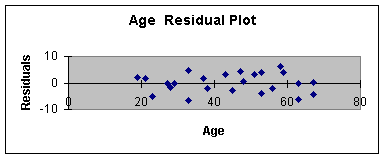Worksheet 3.3.6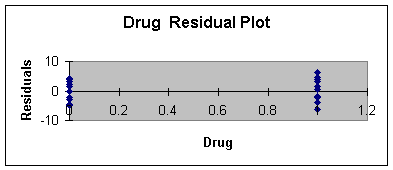The plot of residual or errors against age shows fairly constant variance for all values of Age. Likewise, the errors plotted against drug shows about the same spread for drug = 0 and drug = 1.

Making a Prediction
To predict the effectiveness score for a new drug (drug = 0) administered a 63 year old, we first obtain the point estimate:

Eq. 3.3.4: Effect = 6.2 + 1.03Age + 41.3 Drug - 0.7 Age*Drug;
Effect = 6.2 + 1.03 (63) + 41.3 (0) -0.7 (63*0);
Effect = 6.2 + 64.9 = 71.1

Next, incorporate two times the standard error to get the 95% prediction interval:

Eq. 3.3.5: Effect = 71.1 +/- (2 * 3.877) = 71.1 +/- 7.75

We are 95% confident that a person 63 years of age, using the new drug, will have an effectiveness score between 63.35 and 78.85.

Example Two: Salary Study

Item 1: Enter Data
The second model building example concerns the Salary Study that was introduced in Module Notes 3.2 to illustrate the concepts of curvature, dummy variable, and interaction. I will use that example here to demonstrate how to determine the "best" model - that is, what combination of the the independent variables (quantitative variable, qualitative variable, curvature, and interaction) would best predict faculty salary.

The data that had to be collected includes faculty salary (dependent variable, Y), years of experience (quantitative independent variable, X1), and gender (qualitative independent variable, X2). The data that is created is for the curvature and interaction terms shown in Worksheet 3.3.7.

Worksheet 3.3.7
 Years Years^2 Gender Yrs*Gndr Salary 13 169 1 13 72000 13 169 0 0 68000 10 100 1 10 66000 10 100 0 0 64000 14 196 1 14 64000 8 64 1 8 62000 15 225 1 15 61000 11 121 0 0 60000 9 81 1 9 60000 15 225 0 0 59000 5 25 1 5 59000 12 144 1 12 59000 11 121 1 11 58000 6 36 0 0 57000 7 49 1 7 56000 12 144 0 0 55000 6 36 1 6 55000 9 81 0 0 52000 14 196 0 0 51000 7 49 0 0 50000 3 9 1 3 45000 3 9 0 0 44000 4 16 1 4 44000 4 16 0 0 42000 8 64 0 0 41000 5 25 0 0 34000 2 4 1 2 34000 1 1 1 1 30000 2 4 0 0 25000 1 1 0 0 22000

Item 2: Build Model 1
Worksheet 3.3.8 illustrates the regression summary from using the Regression Analysis Data Analysis Add In.

Worksheet 3.3.8
 SUMMARY OUTPUT Regression Statistics Multiple R 0.901285985 R Square 0.812316427 Adjusted R Square 0.782287055 Standard Error 6034.722055 Observations 30 ANOVA df SS MS F Significance F Regression 4 3940519910 985129977.4 27.05073003 9.23118E-09 Residual 25 910446757.2 36417870.29 Total 29 4850966667 Coefficients Standard Error t Stat P-value Lower 95% Upper 95% Intercept 16652.01465 4452.309573 3.740084642 0.000962747 7482.317863 25821.71144 Years 6723.028442 1122.510607 5.98927832 2.96435E-06 4411.176191 9034.880693 Years^2 -268.1803491 66.43741887 -4.036585912 0.000450987 -405.0106787 -131.3500194 Gender 8619.047619 4637.228193 1.85866368 0.074886968 -931.4959304 18169.59117 Yrs*Gndr -235.7142857 510.0271021 -0.462160314 0.647963296 -1286.13403 814.7054581

As with Example 1, I did not select any of the output Residual or Normal Probability Output options at this time since I am just building the model. Once we have the best model, we can rerun it and produce all of the output options at that time.

First Test: Curvature
Following the decision tree, our first test on Model 1, the full model that includes all of the hypothesized components, is for curvature.

The slope coefficient that we need to use to test curvature is B2. The null and alternate hypotheses to test curvature are:

H0: B2 = 0 (curvature is not important)
Ha: B2 =/= 0 (curvature is important)

The hypothesized model associated with the null hypothesis at this point in the decision tree is Model 2, and the hypothesized model associated with the alternative hypothesis is Model 1:

Model 2 Associates with H0: Salary= B0 + B1 Years + B3 Gender +
B4 Yrs*Gndr

Model 1 Associates with H1: Salary = B0 + B1 Years + B2 Years2 +

B3 Gender + B4 Yrs*Gndr

Since the p-value (0.00045) for the curvature term (Years^2) in Worksheet 3.3.8 is less than alpha of 0.01, reject the null hypothesis, and conclude that curvature is important. This means that Model 1 is a better predictor than Model 2, and at this point, we keep Model 1 as our best model so far. We are at Item 4, A. in the decision tree. Note that the decision tree now tells us to test interaction by examining Model 1 vs. Model 3.

Second Test: Interaction
The only difference between Model 1 and Model 3 is that Model 1 includes interaction, Model 3 does not. Note that both models include curvature since we previously determined that curvature is important in predicting salary. The slope coefficient associated with interaction is B4. The null and alternative hypotheses to test interaction are:

H0: B4 = 0 (interaction is not important)

Ha: B4 =/= 0 (interaction is important)

The hypothesized model associated with the null hypothesis at this point in the decision tree is Model 3, and the hypothesized model associated with the alternative hypothesis is Model 1. Note that both models include curvature.

Model 3 Associates with H0: Salary= B0 + B1 Years + B2 Years2 +
B3 Gender

Model 1 Associates with H1: Salary= B0 + B1 Years + B2 Years2 +

B3 Gender + B4 Yrs*Gndr

Since the p-value (0.647963) for the interaction term (Yrs*Gndr) in Worksheet 3.3.8 is greater than alpha of 0.01, do not reject the null hypothesis, and conclude that interaction is not important. This means that Model 3 is a better predictor than Model 1. Note in the decision tree Item A (2), that if interaction is not important, build Model 3 and test for the importance of the qualitative variable. We need to rerun the regression without the interaction term to create Model 3.

Third Test: Qualitative Variable
To build Model 3, I remove the interaction column and redo the regression analysis. The result is shown in Worksheet 3.3.9.

Worksheet 3.3.9
 Years Years^2 Gender Salary 13 169 1 72000 13 169 0 68000 10 100 1 66000 10 100 0 64000 14 196 1 64000 8 64 1 62000 15 225 1 61000 11 121 0 60000 9 81 1 60000 15 225 0 59000 5 25 1 59000 12 144 1 59000 11 121 1 58000 6 36 0 57000 7 49 1 56000 12 144 0 55000 6 36 1 55000 9 81 0 52000 14 196 0 51000 7 49 0 50000 3 9 1 45000 3 9 0 44000 4 16 1 44000 4 16 0 42000 8 64 0 41000 5 25 0 34000 2 4 1 34000 1 1 1 30000 2 4 0 25000 1 1 0 22000

Next, I run the regression for Model 3. This is shown in Worksheet 3.3.10.

Worksheet 3.3.10
 SUMMARY OUTPUT Regression Statistics Multiple R 0.900395978 R Square 0.810712917 Adjusted R Square 0.7888721 Standard Error 5942.756834 Observations 30 ANOVA df SS MS F Significance F Regression 3 3932741338 1310913779 37.11916585 1.52134E-09 Residual 26 918225328.6 35316358.79 Total 29 4850966667 Coefficients Standard Error t Stat P-value Lower 95% Upper 95% Intercept 17594.87179 3897.092239 4.514871785 0.000120961 9584.278723 25605.46487 Years 6605.171299 1076.50067 6.135780016 1.73624E-06 4392.391031 8817.951568 Years^2 -268.1803491 65.42495602 -4.099052799 0.000360926 -402.6633603 -133.6973378 Gender 6733.333333 2169.987981 3.102935773 0.004577524 2272.856233 11193.81043

To test for the importance of the qualitative variable at this point in the decision tree process (Item 4.A.(2).), we compare Model 3 with Model 4. The only difference between these two models is that Model 3 includes the qualitative variable, Model 4 does not. Note that both models include curvature, and neither model includes interaction, which follows from the testing done so far. The slope coefficient associated with the qualitative variable is B3. The null and alternative hypotheses to test for the qualitative variable are:

H0: B3 = 0 (qualitative variable, gender, is not important)
Ha: B3 =/= 0 (gender is important in predicting salary)

The hypothesized model associated with the null hypothesis at this point in the decision tree is Model 4, and the hypothesized model associated with the alternative hypothesis is Model 3:

Model 4 Associates with H0: Salary= B0 + B1 Years + B2 Years2

Model 3 Associates with H1: Salary = B0 + B1 Years + B2 Years2 +

B3 Gender

Since the p-value (0.004578) for the qualitative term (Gender) in Worksheet 3.3.10 is less than alpha of 0.01, reject the null hypothesis, and conclude that gender is important in predicting salary. This means that Model 3 is a better predictor than Model 4, and at this point, we keep Model 3 as our best model so far. We are at Item 4, A. (2).a. in the decision tree. Note that the decision tree now tells us to stop, we have arrived at our best model. Since curvature is important, there is no need to test the quantitative variable, Years, since Years is needed to make the curvature term. That is why the decision tree said to stop.

Item 5: Assignment 3
Now that we have the best model, rerun the regression and select all of the output options of the Regression Add In dialog box (residual, normal and line fit plots and standardized residuals). You are now ready to interpret the regression coefficients, test practical utility of your model, test statistical utility of your model, evaluate the assumptions, and make a prediction.

The Sample Regression Equation and Interpretation of the Coefficients
Worksheet 3.3.10 provides the coefficients for the sample regression equation:

Eq. 3.3.6: Salary = 17595 + 6605 Years - 268 Years2 +
6733 Gender

Since this model includes gender, we will have two equations, one for male faculty members (X2 = 1) and one for female faculty members (X2 = 0). The equation for male faculty members is:

Eq. 3.3.7: Salary = 14328 + 6605 Years - 268 Years2

The equation for female faculty members is:

Eq. 3.3.8: Salary = 17595 + 6605 Years - 268 Years2

Thus, the slope coefficient on gender, B3 = 6733 in Equation 3.3.6, is the average difference in salary between males and females: males make \$6,733 average more than females. This is a case of gender discrimination since the faculty were similar in all other regards.

The other slope of interest to us is B2, the slope on the curvature term. Its value is -268. About all that we can say from a management interpretation, is that the negative term means we have negative or downward curvature. This means that as experience increases, salary increases but at a decreasing rate. A glimpse at the line fit plot illustrates the above discussion. This plot is shown in Worksheet 3.3.11. Note the two curves, the top being the predicted salary curve for male faculty, the bottom curve for female faculty. The salary curve is typical in a public university as faculty get promoted to associate and then full professor by the 10 - 15 year point, then follow-on raises follow small incremental state yearly increases. What should not be typical is the two curves as that is discrimination if all other factors are the same.

Worksheet 3.3.11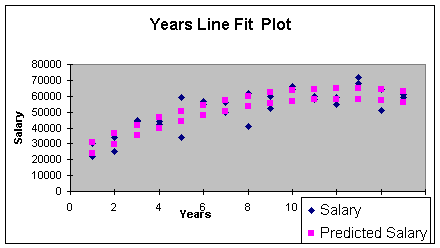Practical Utility
The adjusted R Square is 0.81 meaning that years experience and gender explain 81% of the sample variation in salary in this curvilinear model. This is a high degree of explained variation. The Standard Error of the Model is \$5,943. which is interpreted to be 95% of the actual salaries will be within +/- 2 * \$5,943 or +/- \$11,846 of the predicted salaries. This error is obviously too high for prediction purposes, but if the model was to be used solely to understand the discrimination effect, it might be acceptable. I should add a caution here. You may have noticed that there were just 30 observations in this example. There should have been a minimum of 50. If we used the larger minimum number of observations, the standard error should improve (remember standard errors and standard deviations reduce as you increase sample size to the minimum required).

Statistical Utility
The following hypothesis test is used to determine model utility.

H0: B1 = B2 = B3 = 0 (regression model is not statistically useful)
Ha: At least one B =/= 0 (model is statistically useful)

Since the p-value (1.52E-09) for the F statistic in the Regression Row of the ANOVA table of Worksheet 3.3.10 is less than alpha of 0.01, reject the null hypothesis and conclude that the model is statistically useful.

Assumptions
I examined the standardized residuals and the normal probability plot and found no outliers, indicating that the assumption that the error terms are normally distributed around a mean of zero can be considered met. To determine if we meet the assumptions that the error has constant variance and is independent, we examine the residual plots.

There will be two plots of interest, the plot for the quantitative X and the plot for the qualitative X. You will also get a plot for the curvature term but this is a derived variable and may be ignored. Assignment 3, Step 5, in the Main Module 3 Notes and repeated at the beginning of this note set states exactly what residual plots you need according to which model number is your best model. Worksheet 3.3.12 provides the residual plot for Years, and Worksheet 3.3.13 provides the residual plot for gender.

Worksheet 3.3.12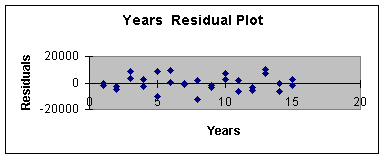Worksheet 3.3.13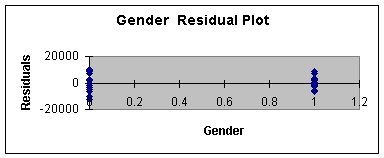The Years Residual Plot shows very little error for 1, 2, 14, and 15 years of experience compared to 5 and 7. As well, the male gender residual is smaller than the female residual. This is a second indication of a small sample size problem. A larger sample size should even out the distribution of the error for all values of the independent variables.

Making a Prediction
To predict the salary for the first female faculty member in our data set (13 years experience), we first obtain the point estimate:

Eq. 3.3.9: Salary = 17595 + 6605 Years - 268 Years2 +
6733 Gender;

Salary = 17595 + 6605 (13) - 268 (13)2 +

6733 (0);

Salary = \$58,168

Next, incorporate the standard error to get a 95% prediction interval:

Eq. 3.3.10: Salary = 58168 +/- (2 * 5943) = 58168 +/- 11886.

So, we are 95% confident that a female faculty member with 13 years experience will make between \$46,282 and \$70,054. As indicated earlier, that is a wide range for prediction purposes. Perhaps more data or stratification of the faculty by years of experience would produce a model with less error.

The error of prediction for this particular observation is actual minus predicted which is 68,000 - 58,186 or \$9,814.

Example Three: External Hour/Asset Study

For the last example, I picked a problem where I knew there was no curvature, interaction or significant quantitative variable - just a simple linear regression. This is the example I used for Module Notes 2.1 and 2.2 .

Item 1: Enter Data
The data for this problem involves a quantitative independent variable, Assets in thousands of dollars; a qualitative independent variable, years of experience (Exp) of the audit team leader; and the dependent variable, External Hours to do an audit. Please note that experience was actually a quantitative variable. I made it into a dummy variable by noting that 1 = team leader with years of experience greater than 5, and 0 = years of experience less than or equal to 5. You can make quantitative variables dummy variables, but not the other way around. Worksheet 3.3.14 provides the data input.

Worksheet 3.3.14
 Assets Assets^2 Exp Asset*Exp ExtHours 3200 10240000 1 3200 700 3000 9000000 1 3000 900 3500 12250000 1 3500 800 4000 16000000 1 4000 900 4700 22090000 1 4700 880 5000 25000000 1 5000 850 6000 36000000 0 0 1000 5500 30250000 0 0 1000 6500 42250000 1 6500 950 6500 42250000 0 0 1100 7000 49000000 0 0 1200 4500 20250000 1 4500 830 7500 56250000 0 0 1100 5750 33062500 1 5750 1100 7250 52562500 0 0 1160 8000 64000000 1 8000 1120 8000 64000000 0 0 1300 8500 72250000 0 0 1400 9000 81000000 1 9000 1500 8500 72250000 0 0 1200

Item 2: Build Model 1
Worksheet 3.3.15 illustrates the regression summary from using the Regression Analysis Data Analysis Add In.

Worksheet 3.3.15
 SUMMARY OUTPUT Regression Statistics Multiple R 0.927239544 R Square 0.859773172 Adjusted R Square 0.822379352 Standard Error 87.51652745 Observations 20 ANOVA df SS MS F Significance F Regression 4 704407.8613 176101.9653 22.99239 2.97E-06 Residual 15 114887.1387 7659.142577 Total 19 819295 Coefficients Standard Error t Stat P-value Lower 95% Upper 95% Intercept 1214.943829 443.3511933 2.740364405 0.015177 269.9625 2159.925 Assets -0.129373898 0.115830849 -1.116920912 0.2816 -0.376262 0.117514 Assets^2 1.66433E-05 7.90665E-06 2.104978726 0.05256 -2.09E-07 3.35E-05 Exp -248.8972384 268.8201043 -0.925887739 0.369162 -821.8741 324.0796 Asset*Exp 0.030084261 0.037500954 0.802226542 0.434948 -0.049847 0.110016

First Test: Curvature
Following the decision tree, our first test on Model 1 is for curvature. The slope coefficient that we need to use to test curvature is B2. The null and alternative hypotheses to test curvature are:

H0: B2 = 0 (curvature is not important)

Ha: B2 =/= 0 (curvature is important)

The hypothesized model associated with the null hypothesis at this point in the decision tree is Model 2, and the hypothesized model associated with the alternative hypothesis is Model 1:

Model 2 Associates with H0: ExtHours = B0 + B1 Assets +
B3 Exp + B4 Assets*Exp

Model 1 Associates with H1: ExtHours = B0 + B1 Assets +

B2 Assets2 + B3 Exp + B4 Assets*Exp

Since the p-value (0.05256) for the curvature term (Years^2) in Worksheet 3.3.14 is greater than 0.01, we fail to reject the null hypothesis and conclude that curvature is not important. At this point, Model 2 is our best model - we are at Item 4.B. in the decision tree. We need to remove curvature from the data set, build Model 2, and prepare for the interaction test.

Second Test: Interaction
Worksheet 3.3.16 illustrates the data with the curvature term removed.

Worksheet 3.3.16
 Assets Exp Asset*Exp ExtHours 3200 1 3200 700 3000 1 3000 900 3500 1 3500 800 4000 1 4000 900 4700 1 4700 880 5000 1 5000 850 6000 0 0 1000 5500 0 0 1000 6500 1 6500 950 6500 0 0 1100 7000 0 0 1200 4500 1 4500 830 7500 0 0 1100 5750 1 5750 1100 7250 0 0 1160 8000 1 8000 1120 8000 0 0 1300 8500 0 0 1400 9000 1 9000 1500 8500 0 0 1200

Next, I run the regression for Model 2. This is shown in Worksheet 3.3.17.

Worksheet 3.3.17
 SUMMARY OUTPUT Regression Statistics Multiple R 0.904627421 R Square 0.818350771 Adjusted R Square 0.784291541 Standard Error 96.44438323 Observations 20 ANOVA df SS MS F Significance F Regression 3 670470.6951 223490.2317 24.02728 3.62E-06 Residual 16 148824.3049 9301.519057 Total 19 819295 Coefficients Standard Error t Stat P-value Lower 95% Upper 95% Intercept 395.5572755 233.8658163 1.691385607 0.110145 -100.216 891.3305 Assets 0.106563467 0.032197854 3.309645011 0.004428 0.038307 0.17482 Exp 57.03551305 249.2139422 0.228861646 0.821876 -471.2743 585.3454 Asset*Exp -0.009424722 0.035777859 -0.263423299 0.795587 -0.08527 0.066421

Step B of the Decision Tree shows that to test interaction, we test Model 2 against Model 5. Similar to before, the only difference between these two Models is that Model 2 includes interaction, Model 5 does not. The slope coefficient associated with interaction is B4. The null and alternative hypotheses to test interaction are:

H0: B4 = 0 (interaction is not important)

Ha: B4 =/= 0 (interaction is important)

The hypothesized model associated with the null hypothesis at this point in the decision tree is Model 5, and the hypothesized model associated with the alternative hypothesis is Model 2. Note that neither model has the curvature term since we already determined that curvature was not important and removed it.

Model 5 Associates with H0: ExtHours = B0 + B1 Assets + B3 Exp

Model 2 Associates with H1: ExtHours = B0 + B1 Assets + B3 Exp

+ B4 Assets*Exp

Since the p-value (0.795587) for the interaction term (Assets*Exp) in Worksheet 3.3.17 is greater than alpha of 0.01, do not reject the null hypothesis, and conclude that interaction is not important. This means that Model 5 is a better predictor than Model 2. Note in the decision tree Step 4.B. (2), that we now must build Model 5 by removing interaction from the data set. We then will test if the qualitative dummy variable is important.

Third Test: Qualitative Variable
Worksheet 3.3.18 illustrates the data with the interaction term removed.

 Assets Exp ExtHours 3200 1 700 3000 1 900 3500 1 800 4000 1 900 4700 1 880 5000 1 850 6000 0 1000 5500 0 1000 6500 1 950 6500 0 1100 7000 0 1200 4500 1 830 7500 0 1100 5750 1 1100 7250 0 1160 8000 1 1120 8000 0 1300 8500 0 1400 9000 1 1500 8500 0 1200

Next, I run the regression for Model 5. This is shown in Worksheet 3.3.19.

 SUMMARY OUTPUT Regression Statistics Multiple R 0.904191882 R Square 0.81756296 Adjusted R Square 0.796099779 Standard Error 93.76747033 Observations 20 ANOVA df SS MS F Significance F Regression 2 669825.2456 334912.6228 38.09142 5.24E-07 Residual 17 149469.7544 8792.338493 Total 19 819295 Coefficients Standard Error t Stat P-value Lower 95% Upper 95% Intercept 450.472272 103.0525338 4.371287684 0.000416 233.0501 667.8944 Assets 0.098930495 0.013649179 7.248091277 1.36E-06 0.070133 0.127728 Exp -7.188434707 50.20635226 -0.143177793 0.887833 -113.1147 98.73786

Step 4.B.(2) of the Decision Tree shows that to test for the importance of the qualitative variable, we test Model 5 against Model 7. Similar to before, the only difference between these two Models is that Model 5 includes the qualitative variable, Model 7 does not. The slope coefficient associated with the qualitative variable is B3. The null and alternative hypotheses to test interaction are:

H0: B3 = 0 (qualitative variable, Exp, is not important)

Ha: B3 =/= 0 (Exp is important in predicting ExtHours)

The hypothesized model associated with the null hypothesis at this point in the decision tree is Model 7, and the hypothesized model associated with the alternative hypothesis is Model 5. Note that neither model has the curvature or interaction terms since we already determined that curvature and interaction were not important and removed them from further model consideration.

Model 7 Associates with H0: ExtHours = B0 + B1 Assets

Model 5 Associates with H1: ExtHours = B0 + B1 Assets + B3 Exp

Since the p-value (0.887833) for the qualitative variable (Exp) in Worksheet 3.3.19 is greater than alpha of 0.01, do not reject the null hypothesis, and conclude that the qualitative variable is not important. This means that Model 7 is a better predictor than Model 5. Note in the decision tree Step 4.B. (2) b., that we now must build Model 7 by removing the qualitative variable from the data set. We then build a new regression analysis and stop (this is for test purposes - if you get to this point in the decision tree, Model 7 will be considered your "best" model so you will have something to analyze for the exam - this is true even if your Model 7 has no statistical utility).

Build Model 7: Simple Linear Regression Model with Quantitative Variable

Worksheet 3.3.20 provides the simple linear regression data for Model 7.

 Assets ExtHours 3200 700 3000 900 3500 800 4000 900 4700 880 5000 850 6000 1000 5500 1000 6500 950 6500 1100 7000 1200 4500 830 7500 1100 5750 1100 7250 1160 8000 1120 8000 1300 8500 1400 9000 1500 8500 1200

Next, we run the regression analysis with residual output, residual plot, line fit plot and normal probability plot. These are used to evaluate practical utility, statistical utility, the assumptions, and to make the prediction.

That's it. I hope these three examples provide a "feel" for model building. You will learn more about it as you build your model for Assignment 3. Remember that these three examples illustrate a Model 2, a Model 3 and a Model 7 - you may have one of the models not illustrated as your best model.

References:

Sheskin, David J. (2000). Handbook of Parametric and Non Parametric Statistical Procedures (2nd. ed.). Boca Raton, FL: CRC Press LLC, Test 28.

Levine, D., Berenson, M. & Stephan, D. (1999). Statistics for Managers Using Microsoft Excel (2nd. ed.). Upper Saddle River, NJ: Prentice-Hall.

Chapter 14 -- Multiple Regression Models

Mason, R., Lind, D. & Marchal, W. (1999). Statistical Techniques in Business and Economics (10th. ed.). Boston: Irwin McGraw Hill.

Chapter 13 -- Multiple Regression and Correlation Analysis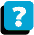About the Course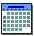Module Schedule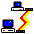WebBoard